# Empirical FormulaPage 2

#### WATCH ALL SLIDES

Caffeine is 49.48% C, 5.15% H, 28.87% N and 16.49% O. What is its empirical formula?

Slide 12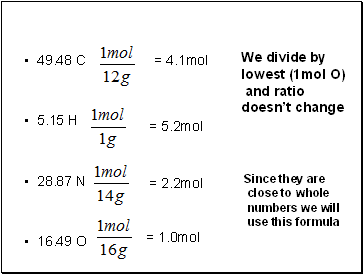49.48 C

5.15 H

28.87 N

16.49 O

= 4.1mol

= 5.2mol

= 2.2mol

= 1.0mol

Since they are

close to whole

numbers we will

use this formula

We divide by

lowest (1mol O)

and ratio

doesn’t change

Slide 13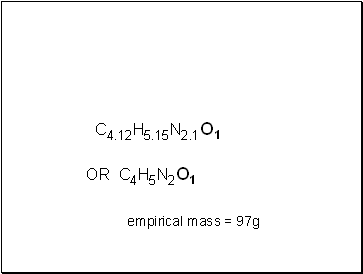C4.12H5.15N2.1O1

empirical mass = 97g

OR C4H5N2O1

Slide 14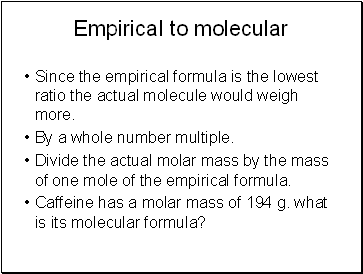## Empirical to molecular

Since the empirical formula is the lowest ratio the actual molecule would weigh more.

By a whole number multiple.

Divide the actual molar mass by the mass of one mole of the empirical formula.

Caffeine has a molar mass of 194 g. what is its molecular formula?

Slide 15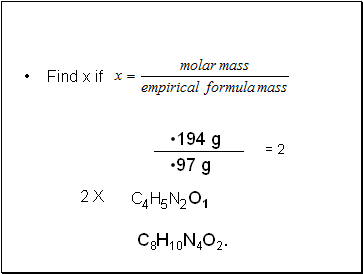Find x if

194 g

97 g

= 2

C4H5N2O1

C8H10N4O2.

2 X

Slide 16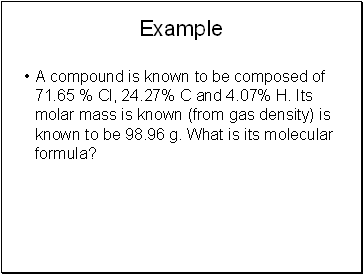Example

A compound is known to be composed of 71.65 % Cl, 24.27% C and 4.07% H. Its molar mass is known (from gas density) is known to be 98.96 g. What is its molecular formula?

Slide 17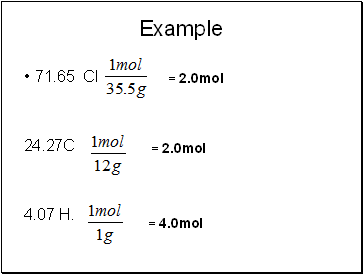Example

71.65 Cl

24.27C

4.07 H.

= 2.0mol

= 2.0mol

= 4.0mol

Slide 18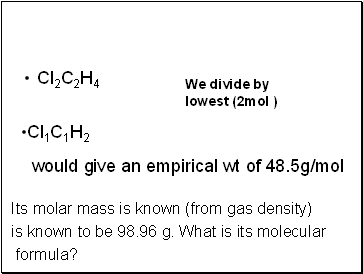Cl2C2H4

Its molar mass is known (from gas density)

is known to be 98.96 g. What is its molecular

formula?

We divide by

lowest (2mol )

Cl1C1H2

would give an empirical wt of 48.5g/mol

Slide 19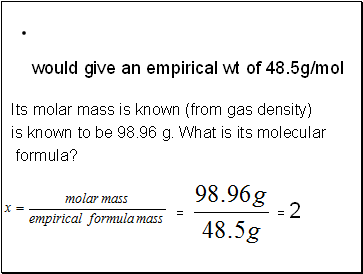Its molar mass is known (from gas density)

is known to be 98.96 g. What is its molecular

formula?

would give an empirical wt of 48.5g/mol

= 2

=

Slide 20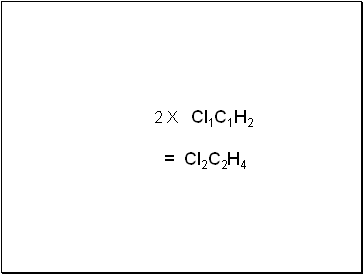2 X Cl1C1H2

= Cl2C2H4

Go to page:
1  2